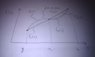# Interpolation Functions and their derivatives

bugatti79
Folks,

How do determine whether the derivative of a quadratic interpolation function ##ax^2+bx+c## is continous/discontinous in the context of the following

We have a a true solution approximated by 2 quadratic interpolation functions ie,

The approximation function
$f_1(x)=ax^2+bx+c, g \le x \le x_1\\ f_1(x)=dx^2+ex+f, x_1 \le x \le h$

See attached my sketch.

Would'nt ##f_1(x)=f_2(x)## and ##f'_1(x)=f'_2(x)## at ##x_1## for the approximation function to be continous?

#### Attachments

•IMAG0063.jpg
13.1 KB · Views: 348

It looks like f and f' are continuous at x1.

Homework Helper
Whether or not you want or need the first deriviative to be continuous depends what the interpolation is used for.

A common notation is "C0-continuous" if the function is continuous but the first derivative is not (except by accident in a special case), and "C1-continuous" if the function and its first derivative are both continuous.

The word "continuous" on its own means "C0-continuous".

Homework Helper
Whether or not you want or need the first deriviative to be continuous depends what the interpolation is used for.
True but there would be little point in using a quadratic to interpolate if we don't want the first derivative to be continuous. The point is that for f(x)= ax^2+ bx+ c, f'(x)= 2ax+b, f''(x)= 2a, a constant. If we only want to match values and don't need "smoothness", we would use piecewise linear functions. If we want to match up second derivatives, we should use piecewise cubics (cubic splines).

bugatti79
Whether or not you want or need the first deriviative to be continuous depends what the interpolation is used for.

A common notation is "C0-continuous" if the function is continuous but the first derivative is not (except by accident in a special case), and "C1-continuous" if the function and its first derivative are both continuous.

The word "continuous" on its own means "C0-continuous".

Well I am referring back to the finite element theory. My query is based on the authors comment as attached.
Why wouldn't the derivative of a second order lagrange interpolation function be continuous as I have shown in my original sketch.

In other words, would'nt ##f'_1(x_1)=f'_2(x_1)## hold and thus the derivatuve is continuous...?

True but there would be little point in using a quadratic to interpolate if we don't want the first derivative to be continuous. The point is that for f(x)= ax^2+ bx+ c, f'(x)= 2ax+b, f''(x)= 2a, a constant. If we only want to match values and don't need "smoothness", we would use piecewise linear functions. If we want to match up second derivatives, we should use piecewise cubics (cubic splines).